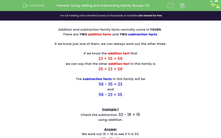# Use Knowledge of Addition and Subtraction Family Groups to Check Subtractions

In this worksheet, students will understand that addition and subtraction are inverses and use this fact to check subtractions.Key stage:  KS 1

Curriculum topic:   Number: Addition and Subtraction

Curriculum subtopic:   Understand Relationship Between Addition and Subtraction

Difficulty level:#### Worksheet Overview

This activity is all about families.No, not that sort of family -  maths families!

Addition and subtraction family facts normally come in fours

There are two addition facts and two subtraction facts.

If we know just one of them, we can always work out the other three.

If we know the addition fact that

23 + 35 = 58

we can say that the other addition fact in this family is

35 + 23 = 58

The subtraction facts in this family will be

58 - 35 = 23

and

58 - 23 = 35Example 1

Check the subtraction 33 - 18 = 15

We work out 15 + 18 to see if it is 33.

15 + 10 + 8

15 + 10 = 25

25 + 8 = 33

which is the related addition fact   15 + 18 = 33

so the subtraction is correct.

Example 2

Check the subtraction 35 - 12 = 25

We work out 25 + 12 to see if it is 35.

25 + 10 + 2

25 + 10 = 35

35 + 2 = 37  and not 35

so the subtraction is wrong.

Do you get the idea? Let's have a go at some questions.

### What is EdPlace?

We're your National Curriculum aligned online education content provider helping each child succeed in English, maths and science from year 1 to GCSE. With an EdPlace account you’ll be able to track and measure progress, helping each child achieve their best. We build confidence and attainment by personalising each child’s learning at a level that suits them.

Get started